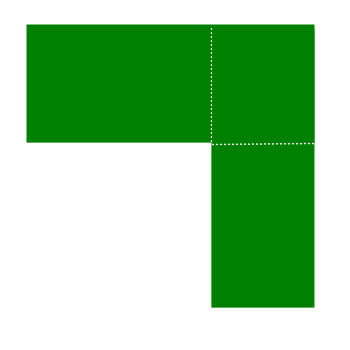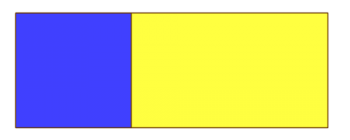Games
Problems
Go Pro!

# Rectangle and Square

Pro Problems > Math > Geometry > Rectangles and Squares

## Rectangle and Square

The length of a rectangle is 3 times its width. If the length is decreased by 3, and the width is increased by 11, the resulting figure is a square. What is the area of the original rectangle?

Presentation mode
Problem by allie

## Solution

In order to make it feasible for teachers to use these problems in their classwork, no solutions are publicly visible, so students cannot simply look up the answers. If you would like to view the solutions to these problems, you must have a Virtual Classroom subscription.Assign this problem

## Similar Problems

### On the Edge

Square X has sides of length n units. Its interior is filled with squares of side length 1 unit.

These same unit squares could be taken from the interior of X and placed along the edges of square Y, so that all the unit squares are on the exterior of square Y, with a single edge of each unit square against an edge of Y, and no part of any edge of Y does not touch a unit square.

There are two more squares, which are non-overlapping: H and K. The length of a side of K is 4 less than the length of a side of H. All the unit squares could be placed on the interior of squares H and K, with at least one edge of each unit square against an edge of either H or K, and such that no part of any edge of H or K does not touch a unit square.

If the sides of square H are 5 units shorter than the sides of square Y, how many unit squares are there?

### Decreasing Width

The area of a rectangle is 288 square inches. If the width is decreased by 20, the new area would be 168. What is the perimeter of the original rectangle?

### Overlapped RectanglesTwo rectangles are overlapped on a corner as shown in the image. The overlapped region is a square. The length of one rectangle is twice the length of the other, and its width is half the width of the other.

If we consider the rectangles as a single geometric figure, its outer perimeter is 768 units, and its area is 8415 square units.

The perimeter of the first rectangle is 312 units.

Find the area of the overlapped square, given that all the side measures are integers.

### Splitting the Field

The perimeter of a field is 2240 feet. The field is split down the middle, parallel to the shorter dimension. The perimeter of each half-field is 1520 feet. What is the shorter dimension of the field?

### Field Area

Farmer Bob has a rectangular field which he can fence in with 5,000 feet of fencing material. The field is 4 times as long as it is wide.

What is the area of the field?

### Building a Room

I want to build a room which has width 6 feet less than twice its length. The area of the room will be 216 square feet. What is the width of the room?

### The Farmer in the Dell

Farmer Dell has a field that is shaped like a letter L, and he wants to divide it into three parts, as shown below:The region in the upper right corner has a perimeter of 140 yards. The regions in the upper left corner and the lower right corner both have perimeters of 180 yards. He originally had enough fencing to enclose the two top regions, and now he needs an extra 140 feet of fencing to complete the job.

What is the total area of his field?

### Stretching Rectangle

The height of a rectangle is decreased by two inches, and the width is increased by three inches. The new rectangle’s area is 14 square inches more than the original rectangle’s area.  If the dimensions of the rectangle are integers, what is the smallest possible area of the original rectangle?

### Area, Perimeter, Diagonal

The area of a rectangle is 192 square feet, and its perimeter is 54 feet. How many feet long is the diagonal of the rectangle?

### Blue Square, Yellow Rectangle

In the diagram shown, the blue square and the yellow rectangle share a side.The area of yellow rectangle is 96 square units more than the area of the blue square.

The combined area of the two figures is 384 square units.

How long is the common side of the rectangle and the square?

Rectangle Diagonal, Perimeter and Lengths

# Featured Resources on This Site

Create Christmas ornaments and more
Print fun mazes in many different shapes

# Blogs on This SiteReviews and book lists - books we love!The site administrator fields questions from visitors.Like us on Facebook to get updates about new resources